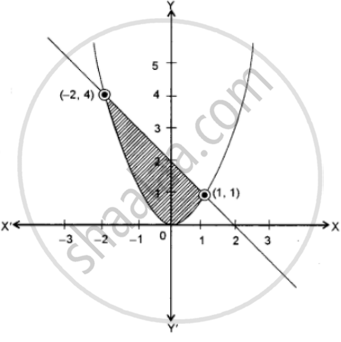# Draw a Rough Sketch and Find the Area Bounded by the Curve X2 = Y and X + Y = 2. - Mathematics

Sum

Draw a rough sketch and find the area bounded by the curve x2 = y and x + y = 2.

#### SolutionThe given curves are: x2 = y

Which is an upward parabola with vertex at origin

And line x + y = 2 ⇒ y = 2 – x

x2 = 2 – x

⇒ x2 + x – 2 = 0

⇒ (x + 2)(x – 1) = 0

⇒ x = -2 and x = 1

Now, y = 2-(-2) = 4

and y = 2 – 1 ⇒ y = 1

⇒ y = 4 and y = 1

Thus, the points of intersection are (-2, 4) and (1, 1)

The required area of the shaded region

= int_-2^1 (2 - "x") "dx" - int_-2^1 "x"^2 "dx"

= |2"x" - "x"^2/2|_-2^1 - |"x"^3/3|_-2^1

= 2 - 1/2 + 4 + 4/2 - 1/3 - 8/3

= (12 - 3 + 24 + 12 - 2 - 16)/6

= 9/2 sq.units

Concept: Area Under Simple Curves
Is there an error in this question or solution?